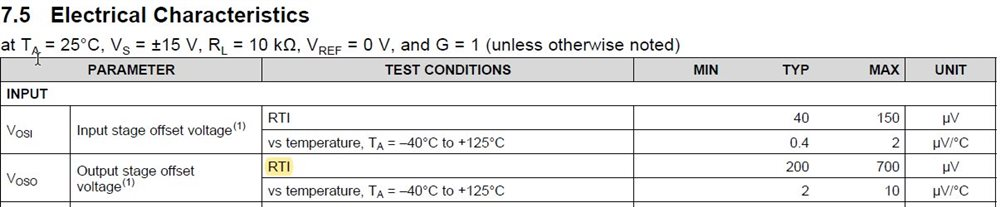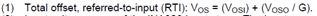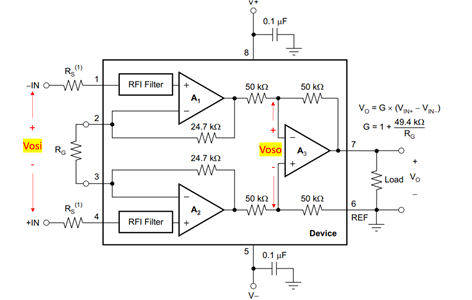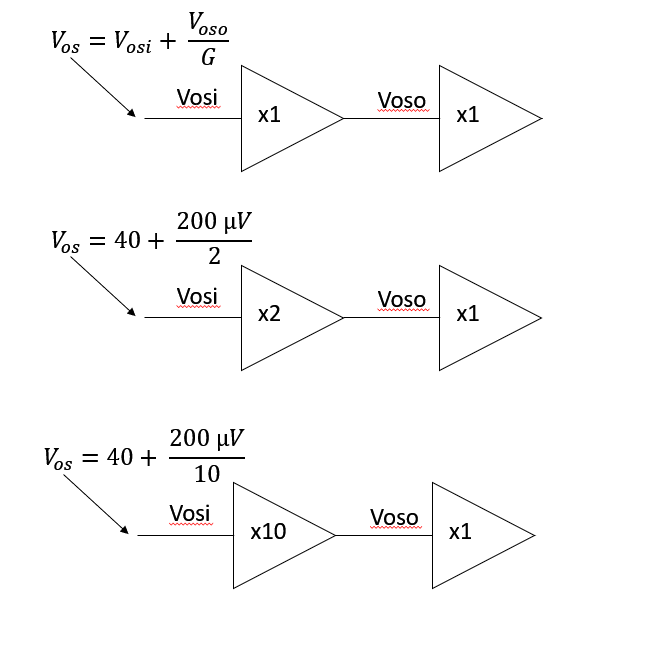If you have a related question, please click the "Ask a related question" button in the top right corner. The newly created question will be automatically linked to this question.

# INA826: Output stage offset referred to input or not?

Part Number: INA826

Hello,

The datasheet states in the Characteristics table, that the Output offset is referred to input (RTI).
so for the output offset, I would multiply the 200uV with the selected gain.but in the formula, that is referenced by the footnot (1), the Voso has to be divided by the selected gain.
This implies, that it is referred to output.Am i missing something?

• Hi Sebastian,

The electrical characteristics table states at the top that the measurement of 200 uV is made in a gain of 1, G = 1. In the formula G = 1.

I hope this helps clarify. Let me know if I can be of further assistance.

Best Regards,

Chris Featherstone

• Hi Chris,

I am just a little less confuse...I think you say, because the general test condition for the 200u is with G=1, the value is correct when referred to Input? Although it is meant as RTO?

So when I apply a gain other than 1 i have to multiply the 200u with the general condition G=1 for RTO and then apply the formula by dividing it with my selected gain?

Or can you give me a calculation example, if I would have lets say a gain of G=2.
Best for both "sides" meaning RTI and RTO.

Regards Sebastian

• Hey Sebastian,

The Vosi refers to the input offset voltage of the input stage op amp block. Voso refers to the input offset voltage of the output stage block in the INA. I made a high level block diagram model to hopefully help simplify the model. The examples below are for a gain of 2 and 10. Let me know if this helps clarify and if you have any further questions.Best Regards,

Chris Featherstone

• Hi Chris,

thank you.
Now it is clear.

Maybe you can think of removing the Testcondition entry of "RTI" in Voso.